Courses

# Physics Test 5 - Magnetic Effect, Magnetism, Electromagnetic Induction And AC

## 45 Questions MCQ Test NEET Mock Test Series & Past Year Papers | Physics Test 5 - Magnetic Effect, Magnetism, Electromagnetic Induction And AC

Description
This mock test of Physics Test 5 - Magnetic Effect, Magnetism, Electromagnetic Induction And AC for NEET helps you for every NEET entrance exam. This contains 45 Multiple Choice Questions for NEET Physics Test 5 - Magnetic Effect, Magnetism, Electromagnetic Induction And AC (mcq) to study with solutions a complete question bank. The solved questions answers in this Physics Test 5 - Magnetic Effect, Magnetism, Electromagnetic Induction And AC quiz give you a good mix of easy questions and tough questions. NEET students definitely take this Physics Test 5 - Magnetic Effect, Magnetism, Electromagnetic Induction And AC exercise for a better result in the exam. You can find other Physics Test 5 - Magnetic Effect, Magnetism, Electromagnetic Induction And AC extra questions, long questions & short questions for NEET on EduRev as well by searching above.
QUESTION: 1

Solution:
QUESTION: 2

Solution:
QUESTION: 3

### An electron is travelling along the x-direction. It encounters a magnetic field in the y-direction. Its subsequent motion will be

Solution:
QUESTION: 4
The magnetic Resonance Imaging (MRI) is based on the phenomenon of
Solution:
QUESTION: 5

A circular coil of radius R carries an electric current. The magnetic field due to the coil a point on the axis of the coil located at a distance r from the centre of the coil, such that r >> R, varies as

Solution:

When r >> R Magnetic field at a point on the axis of a loop is given by B = (mu /2 * pi) (AI/ r³)Hence B is proportional to 1/r³ Hence Correct option is D.

QUESTION: 6

A cyclotron is operating at a frequency of 12 x 106 Hz. Mass of deuteron is 3.3 x 10 -27 kg and charge on deuteron is  1.6 x 10 -19 induction of the necessary magnetic field is

Solution:
QUESTION: 7

The strength of the transverse magnetic field required to bend photoelectrons of maximum kinetic energy with in a circle of radius 50 cm when light of wavelength 4000Å is incident on barium emitter is (work function of barium is 2.5 eV)

Solution:
QUESTION: 8

Two equal electric currents are flowing in wires perpendicular to each other as shown in figure. AB and CD are lines perpendicular to each other and symmetrically placed with respect to currents. Then the resultant magnetic field will be zero on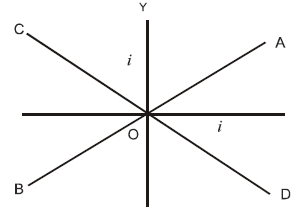Solution:

Applying right hand grip rule and considering AB, the direction of magnetic field due to one current is upwards and that due to other is downwards. Both the magnetic fields cancel out each other, and the resultant magnetic field is zero. Considering CD and applying right hand grip rule for the two currents, the direction of magnetic field is in the same direction in both the cases giving non-zero resultant

QUESTION: 9
A straight wire of diameter 0.5 mm carrying a current of 1 A is replaced by another wire of 1 mm diameter carrying the same current. The strength of magnetic field far away is
Solution:
QUESTION: 10

The semicircular portion (radius R) of a wire carrying current i is placed in a uniform magnetic field B. The magnetic field is perpendicular to the plane of the circle. The magnetic of the force on the wire is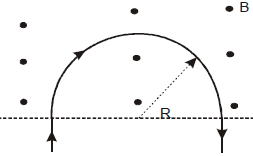Solution:
QUESTION: 11

A & B are two concentric circular conductors of centre O and carrying currents i1 & i2 as shown in the diagram. If ratio of their radii is 1:2 and ratio of the flux densities at O due to A and B is 1:3, then the value of i1 & i2 will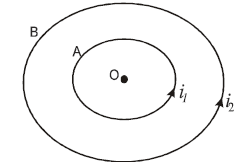Solution:
QUESTION: 12
Domain formation is the necessary feature of
Solution:
QUESTION: 13

A magnet 10 cm long and having a pole strength 2 A-m is deflected through 30º from the magnetic meridian. The horizontal component of earth’s induction is  0.32 x 10 -4T then the value of deflecting couple is

Solution:
QUESTION: 14

A spectrum of iron of permeability 8 x 10 -3 Wb / A-m  is placed in a magnetic field of strength 160 A/m. Then magnetic induction in this iron is

Solution:
QUESTION: 15

Two magnets each of magnetic moment M are placed so as to form a cross at right angles to each other. The magnetic moment of the system will be

Solution:
QUESTION: 16

At a certain place, the horizontal component of earth’s magnetic field is √3 times of its vertical component, the angle of dip at that place is

Solution:
QUESTION: 17

The work done in turning a magnet of magnetic moment M by an angle of 90ºfrom the meridian is n times the corresponding work done to turn it through an angle of 60º. The value of n is given by

Solution:
QUESTION: 18

The time of vibration of a dip needle vibrating in the vertical plane in the magnetic meridian is 3s.When the same magnetic needle is made to vibrate in the horizontal plane, the time is vibration is again 3s. Then angle of dip will be

Solution:
QUESTION: 19

A magnet makes 30 oscillations per minute in the earth’s magnetic field. If the magnetic field is doubled. The period of oscillation of magnet is

Solution:
QUESTION: 20

A moving conductor coil produces an induced emf. This is in accordance with

Solution:
QUESTION: 21

The varying current in a coil changes from 10 A to zero in 0.5 s. If the average emf induced in th coil is 220 V, then the self-inductance of the coil will be

Solution:
QUESTION: 22

Magnetic flux in a circuit containing a coil of resistance 2Ωchanges from 2.0 Wb to 10 Wb in 0.2 s. The charge passed through the coil in this time is

Solution:
QUESTION: 23
A metallic square loop ABCD is moving in its own plane with velocity v in a uniform magnetic field perpendicular to its plane as shown in the figure. An electric field is induced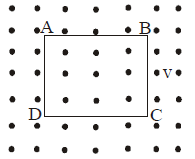Solution:
QUESTION: 24

In the circuit shown in the figure, what is the value of I1 just after pressing the key K?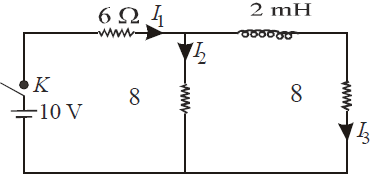Solution:
QUESTION: 25

A uniform but time-varying magnetic field B(t) exists in a cylindrical region of radius a and is directed into the plane of the paper, as shown. The magnitude of the induced electric field at point P at a distance r from the centre of the circular region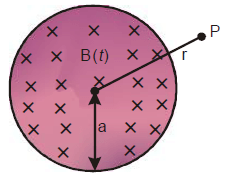Solution:
QUESTION: 26

A conducting rod AC of length 4I is rotated about a point O in a uniform mangetic field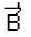directed into the paper. AO =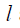and OC = 3. Then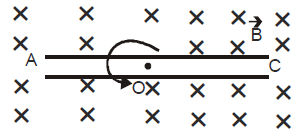Solution: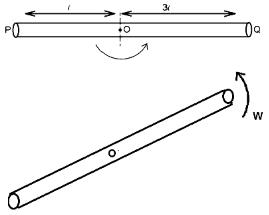Potential difference between point O & P is VO – VA.

Potential difference between point O & Q is VO – VC

Potential difference between point P & Q is

VA – VC = (VO – VC) – (VO – VA) (1)

Now for a rotating rod emf = BVℓ

Consider a small element of length dℓ angular velocity = ω = (V/ℓ) hence

dE = Bωℓ dℓ

∴ Total emf = O Bωℓ dℓ = [{Bωℓ2}/{2}]

∴ VO – VA = [{Bωℓ2}/{2}]

VO – VC = [{Bω(3ℓ)2}/ {2}]

From (1),
VA – VC = (9/2) Bωℓ2 – [{Bωℓ2} /{2}] = 4 Bωℓ2.

QUESTION: 27

Switch S of the circuit shown in figure is closed at t = 0. If e denotes the induced emf in L and i , the current flowing through the circuit at time t, which of the following graphs is correct?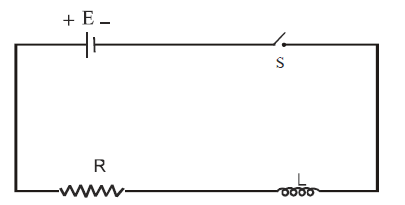Solution:
QUESTION: 28
A device for generating and alternating current of a desired frequency is known as
Solution:
QUESTION: 29

In an AC circuit containing only capacitance,the current

Solution:
QUESTION: 30

The rms value of emf of a saw totothed AC of peak voltage 60 volt is

Solution:
QUESTION: 31
In an ideal parallel L-C circuit, the capacitor is charged by connecting it to a DC source which is then disconnected. The current in the circuit
Solution:
QUESTION: 32

The rms value of AC voltage is  v 3+4 sin (100 πt )

Solution:
QUESTION: 33

Two coils C1 & C2 are placed adjacent to each other, if current is changed in coil C1 at a rate of 5A/sec, the emf induced in coil C2 is 20mV. What emf will be induced in 7C1 if the current is changed in Coil C2 at a rate of 7 A/Sec (Assume there is no change in orientation or position of the coils)

Solution:
QUESTION: 34

A magnetic needle is kept in a non uniform magnetic field. It experiences

Solution:
QUESTION: 35

A Cu ring is suspended by a thread in a vertical plane. The north pole of a magnet is brought near the ring in horizontal direction as shown in figure. What will be effect on the ring ?

Solution:
QUESTION: 36

An emf  E = 4 Cos 1000 t volt is applied to an L-R circuit of inductance 3 mH and resistance 4 Ω. The amplitude of the current in the circuit is

Solution:
QUESTION: 37

Direction : Read the following question and choose

A. If both Assertion and Reason are correct and reason is the correct explanation of assertion

B. If both Assertion and Reason are true, but Reason is not correct explanation of the Assertion

C. If Assertion is true, but the Reason is false

D. If Assertion is false, but the Reason is true

E. If both assertion and reason are false

Assertion : A.C. is more dangerous than D.C.

Reason: The peak value of A.C. is 41.4% higher than the D.C

Solution:
QUESTION: 38

Direction : Read the following question and choose

A. If both Assertion and Reason are correct and reason is the correct explanation of assertion

B. If both Assertion and Reason are true, but Reason is not correct explanation of the Assertion

C. If Assertion is true, but the Reason is false

D. If Assertion is false, but the Reason is true

E. If both assertion and reason are false

Assertion: Self Inductance is also known as inertia of electricity.

Reason : Self Inductance is the property of Coil due to which the coil opposes the flow current through it

Solution:
QUESTION: 39

Direction : Read the following question and choose

A. If both Assertion and Reason are correct and reason is the correct explanation of assertion

B. If both Assertion and Reason are true, but Reason is not correct explanation of the Assertion

C. If Assertion is true, but the Reason is false

D. If Assertion is false, but the Reason is true

E. If both assertion and reason are false
Assertion: In the phenomenon of mutual induction self induction of each of the coils persists
Reason: Self induction arises when strength of current in one coil changes. In this case, current may be changing in both the individual coils

Solution:
QUESTION: 40

Direction : Read the following question and choose

A. If both Assertion and Reason are correct and reason is the correct explanation of assertion

B. If both Assertion and Reason are true, but Reason is not correct explanation of the Assertion

C. If Assertion is true, but the Reason is false

D. If Assertion is false, but the Reason is true

E. If both assertion and reason are false
Assertion: When number of turns in a coil is doubled, coefficient of self-inductance of the coil becomes 4 times
Reason : This is because L ∞ N2

Solution:
QUESTION: 41

Direction : Read the following question and choose

A. If both Assertion and Reason are correct and reason is the correct explanation of assertion

B. If both Assertion and Reason are true, but Reason is not correct explanation of the Assertion

C. If Assertion is true, but the Reason is false

D. If Assertion is false, but the Reason is true

E. If both assertion and reason are false
Assertion: It is more difficult to push a magnet into a coil with more loops
Reason : This is because emf induced in each current loop resists the motion of the magnet

Solution:
QUESTION: 42

Direction : Read the following question and choose

A. If both Assertion and Reason are correct and reason is the correct explanation of assertion

B. If both Assertion and Reason are true, but Reason is not correct explanation of the Assertion

C. If Assertion is true, but the Reason is false

D. If Assertion is false, but the Reason is true

E. If both assertion and reason are false
Assertion :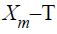graph for a diamagnetic material is a straight line parallel to T-axis

Reason : This is because susceptibility of a diamagnetic material is not affected by temperature

Solution:
QUESTION: 43

Direction : Read the following question and choose

A. If both Assertion and Reason are correct and reason is the correct explanation of assertion

B. If both Assertion and Reason are true, but Reason is not correct explanation of the Assertion

C. If Assertion is true, but the Reason is false

D. If Assertion is false, but the Reason is true

E. If both assertion and reason are false
Assertion : In an electric field, an electron beam perpendicular to field is deflected along a parabola, and in a magnetic field, the electron path perpendicular to the field will be circular
Reason: In electric field, force is perpendicular to path and along the field.In magnetic field, force is perpendicular to both, the magnetic field and path of beam

Solution:
QUESTION: 44

Direction : Read the following question and choose

A. If both Assertion and Reason are correct and reason is the correct explanation of assertion

B. If both Assertion and Reason are true, but Reason is not correct explanation of the Assertion

C. If Assertion is true, but the Reason is false

D. If Assertion is false, but the Reason is true

E. If both assertion and reason are false
Assertion: The energy of charged particle moving in a uniform magnetic field does not change
Reason: Work done by the magnetic field on the charge is zero

Solution:
QUESTION: 45

Direction : Read the following question and choose

A. If both Assertion and Reason are correct and reason is the correct explanation of assertion

B. If both Assertion and Reason are true, but Reason is not correct explanation of the Assertion

C. If Assertion is true, but the Reason is false

D. If Assertion is false, but the Reason is true

E. If both assertion and reason are false
Assertion: A linear solenoid carrying current is equivalent to a bar magnet
Reason: The magnetic field lines of both are same

Solution: